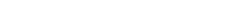# 2、one-hot编码带来的问题

FM(Factorization Machine)主要是为了解决数据稀疏的情况下，特征怎样组合的问题。已一个广告分类的问题为例，根据用户与广告位的一些特征，来预测用户是否会点击广告。数据如下：(本例来自美团技术团队分享的paper)one-hot编码带来的另一个问题是特征空间变大。同样以上面淘宝上的item为例，将item进行one-hot编码以后，样本空间有一个categorical变为了百万维的数值特征，特征空间一下子暴增一百万。所以大厂动不动上亿维度，就是这么来的。

# 3、对特征进行组合# 4、FM求解# 5、tensorflow代码详解

https://github.com/princewen/tensorflow_practice/tree/master/recommendation-FM-demo。``csr_matrix((data, indices, indptr)``

``def vectorize_dic(dic,ix=None,p=None,n=0,g=0):"""    dic -- dictionary of feature lists. Keys are the name of features    ix -- index generator (default None)    p -- dimension of featrure space (number of columns in the sparse matrix) (default None)    """ifix==None:        ix = dict()    nz = n * g    col_ix = np.empty(nz,dtype = int)    i =0fork,lisindic.items():fortinrange(len(lis)):            ix[str(lis[t]) + str(k)] = ix.get(str(lis[t]) + str(k),0) +1col_ix[i+t*g] = ix[str(lis[t]) + str(k)]        i +=1row_ix = np.repeat(np.arange(0,n),g)    data = np.ones(nz)ifp ==None:        p = len(ix)    ixx = np.where(col_ix < p)returncsr.csr_matrix((data[ixx],(row_ix[ixx],col_ix[ixx])),shape=(n,p)),ixcols = ['user','item','rating','timestamp']train = pd.read_csv('data/ua.base',delimiter='t',names = cols)test = pd.read_csv('data/ua.test',delimiter='t',names = cols)x_train,ix = vectorize_dic({'users':train['user'].values,'items':train['item'].values},n=len(train.index),g=2)x_test,ix = vectorize_dic({'users':test['user'].values,'items':test['item'].values},ix,x_train.shape,n=len(test.index),g=2)y_train = train['rating'].valuesy_test = test['rating'].valuesx_train = x_train.todense()x_test = x_test.todense()````n,p = x_train.shapek = 10x = tf.placeholder('float',[None,p])y = tf.placeholder('float',[None,1])w0 = tf.Variable(tf.zeros())w = tf.Variable(tf.zeros([p]))v = tf.Variable(tf.random_normal([k,p],mean=0,stddev=0.01))#y_hat = tf.Variable(tf.zeros([n,1]))linear_terms = tf.add(w0,tf.reduce_sum(tf.multiply(w,x),1,keep_dims=True))# n * 1pair_interactions =0.5* tf.reduce_sum(    tf.subtract(        tf.pow(            tf.matmul(x,tf.transpose(v)),2),        tf.matmul(tf.pow(x,2),tf.transpose(tf.pow(v,2)))    ),axis =1, keep_dims=True)y_hat = tf.add(linear_terms,pair_interactions)``

``lambda_w = tf.constant(0.001,name='lambda_w')lambda_v = tf.constant(0.001,name='lambda_v')l2_norm = tf.reduce_sum(    tf.add(        tf.multiply(lambda_w,tf.pow(w,2)),        tf.multiply(lambda_v,tf.pow(v,2))    ))error = tf.reduce_mean(tf.square(y-y_hat))loss = tf.add(error,l2_norm)train_op = tf.train.GradientDescentOptimizer(learning_rate=0.01).minimize(loss)``

``epochs = 10batch_size = 1000# Launch the graphinit = tf.global_variables_initializer()with tf.Session() as sess:    sess.run(init)    for epoch in tqdm(range(epochs), unit='epoch'):        perm = np.random.permutation(x_train.shape)# iterate over batchesforbX, bYinbatcher(x_train[perm], y_train[perm], batch_size):            _,t = sess.run([train_op,loss], feed_dict={x: bX.reshape(-1, p), y: bY.reshape(-1,1)})            print(t)    errors = []forbX, bYinbatcher(x_test, y_test):        errors.append(sess.run(error, feed_dict={x: bX.reshape(-1, p), y: bY.reshape(-1,1)}))        print(errors)    RMSE = np.sqrt(np.array(errors).mean())print(RMSE)``

# 参考文章：

1、http://blog.csdn.net/bitcarmanlee/article/details/52143909

2、https://blog.csdn.net/u012871493/article/details/51593451